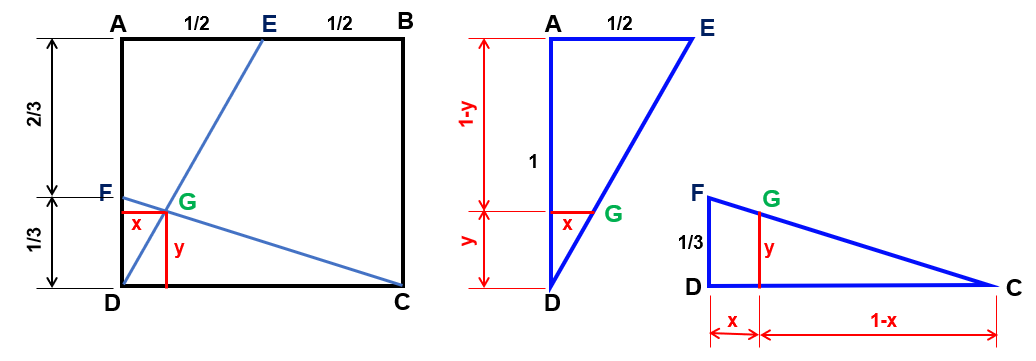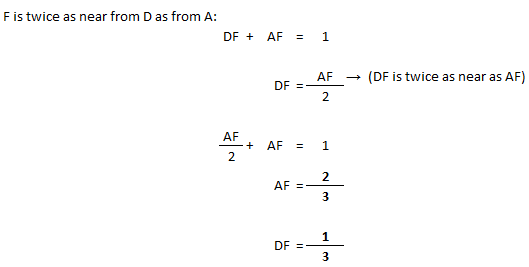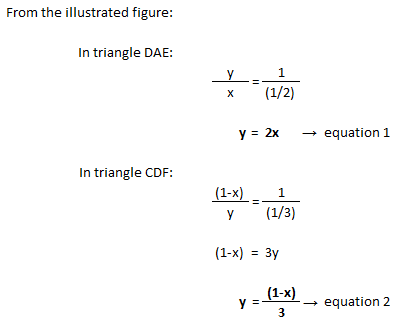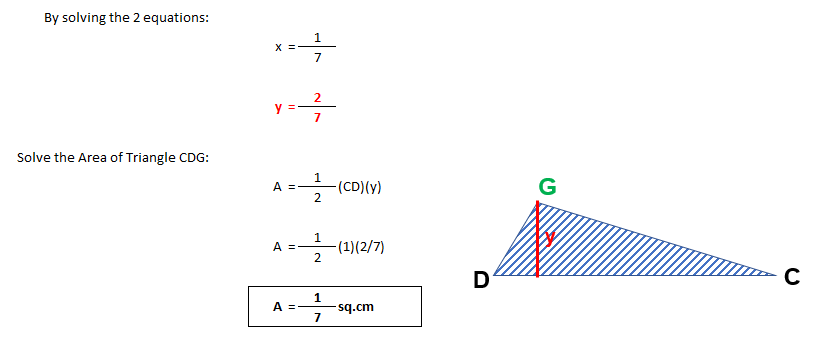# Area of a triangle

8 posts / 0 new
Engr JaydeeArea of a triangle

In square $ABCD$, $E$ is the midpoint of side $\overline{AB}$ and $F$ is a point of side $\overline{AD}$ such that $F$ is twice as near from $D$ as from $A$. $G$ is the intersection of the line segments $\overline{DE}$ and $\overline{CF}$. If $AB = 1\text{ cm}$, find the area of $\triangle CDG$.

There are several ways to solve this problem, please show your solutions.

Rate:
alidoandrei28

I used analytic geometry to get the equation of the lines DE and CF. Thus,

DE: (0,0) (0.5,0.5)
equation: y = x

FC: (0,1/3) (1,0)
equation: y = 1/3 - (1/3)x

Then, I get the point of intersection; (0.25,0.25)

After that, I used integral calculus to solve for the area using horizontal strip.

\$[(1-3y) - y]dy from 0 to 0.25 = 0.125

Thus, the area of triangle CDG = 0.125 cm^2

-please correct me if I'm wrong. Thanks!

Rate:
Angkol-Awin

alidoandrei28

Please Check again your coordinates of line DE: (0,0) , must be (0.5,1).

Rate:
Engr JaydeeHi alidoandrei28,

The coordinates of E should be (1/2, 1) instead of (1/2, 1/2). The rest of the solutions will be correct if this is considered. :)

Rate:

Yes. Using the area of triangle for 2 sides and included angle, or Heron's formula for three sides. The answer is 0.14 cm2.
Solution:
Square ABCD, given 1cm for each side
Line EB=Line EA=1/2cm
Line FD=1/3cm
Line AF=2/3cm
Right Triangle BCE: By Pythagorean theorem, Line CE=square root of 1.25 or 1.12 (2 decimal place). Using tangent function, the other 2 angle (C & E) could be determined and that is 26.6 deg. and 63.4 deg. respectively.
Right Triangle ADE: the same procedure as in triangle BCE to establish angle E & D.
Right Triangle CDF: the same procedure as in triangle BCE & ADE to establish angle C.
Triangle CEG: Angle E=180-63.4-63.4= 53.2; Angle C=90-26.6-18.4= 45; Angle G=180-98.2= 81.8; Now using sine law, line CG & EG could be solved.
Triangle CDG: 3 interior angle already been established (Angle C, D & G = 18.4, 63.4 & 98.2 respectively).
Hence, area of triangle CDG could be solved using area of triangle as mentioned above.

Rate:
Engr JaydeeThe answer and solution is correct, although I think it can shortened further.

You can find angles EDC and FCD by using the tangent function, and then proceed to getting the area of triangle CDG using the formula $$A=\frac{a^2 \sin \beta \sin \gamma}{2 \sin \alpha}$$

Rate:
Angkol-AwinRate:
Engr JaydeeYes, this is correct. :)

Rate: